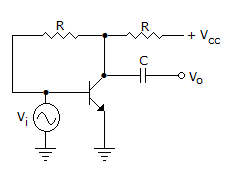# Electronics and Communication Engineering - Analog Electronics

1.

Which op-amp circuit uses a resistance in series with input and capacitor in feedback path?

 A. Differentiating amplifier B. Integrating amplifier C. Logarithmic amplifier D. Exponential amplifier

Explanation:

No answer description available for this question. Let us discuss.

2.

Saturation of iron core causes distortion.

 A. True B. False

Explanation:

No answer description available for this question. Let us discuss.

3.

Which of the following is non-sinusoidal oscillator?

 A. Multivibrator B. Relaxation oscillator using UJT C. Relaxation oscillator using tunnel diode D. Any of the above

Explanation:

No answer description available for this question. Let us discuss.

4.

Low gain-bandwidth product of a JFET is due to

 A. reduced number of holes B. junction capacitive effects C. low degree of isolation between input and output D. all of the above

Explanation:

No answer description available for this question. Let us discuss.

5.

The circuit of the figure is an example of feedback of the following typeA. current series B. current shunt C. voltage series D. voltage shunt